# Community Detection in Graphs¶



### Thomas Bonald¶

Ongoing work with Marc Lelarge and Alexandre Hollocou

# Motivation¶

Graphs are everywhere

• Infrastructure (roads, airlines, Internet,...)
• Information (Web, Wikipedia,...)
• Biology (brain, protein interaction, ...)

How to extract information from these graphs?

# Community detection¶

Real-life graphs are organized in communities ($\approx$ dense groups of nodes)

We seek to infer these communites

Applications:

• Search engines
• Content recommandation
• Data vizualisation
• Classification
• ...

# Local community detection¶

Most existing algorithms give a partition of the whole graph

We are interested in algorithms revealing the local structure around some target nodes (the seed set)

These local communities are typically:

• centered
• hierarchical
• overlapping

# Outline¶

1. Background
2. Ranking
3. Quality metrics
4. Live examples
5. Future work

# How do graphs look like?¶

Most graphs are big, scale-free and small-world

$$\begin{array}{l|c|c} & \text{DBLP} & \text{Wikipedia} \\ \hline \text{# nodes} & 1.2\text{M} & 4.2\text{M} \\ \text{# edges} & 5.3\text{M} & 101\text{M} \\ \text{average degree} & 8 & 24\\ \text{standard deviation} & 21 & 48\\ \text{3-hop population} & 91\% & 20\% \text{(out) } 98\% \text{(in)}\\ \end{array}$$

Karinthy 1929, Milgram 1967

# Modularity¶

Given a partition of the (undirected) graph, the modularity is defined by $$Q = \frac 1 {2m}\sum_{i,j}A_{ij}\delta_{ij} - \frac 1{(2m)^2} \sum_{i,j}d_{i}d_j\delta_{ij}$$ where

• $A$ is the adjacency matrix
• $d = A1$ is the vector of degrees
• $\delta_{ij}=1$ if $i$ and $j$ are in the same community

# Example¶

In :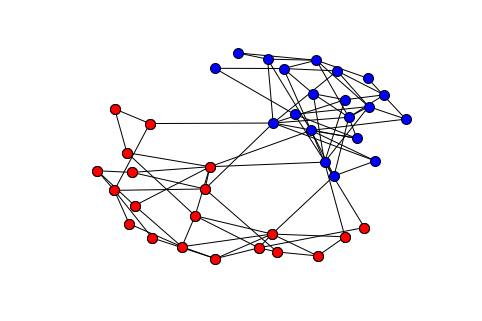In :
modularity(A,C)

Out:
0.39998548410509505

# Modularity of weighted graphs¶

$$Q = \frac 1 {w^T1}\sum_{i,j}A_{ij}\delta_{ij} - \frac 1{(w^T1)^2} \sum_{i,j}w_{i}w_j\delta_{ij}$$

where

• $A$ is the weighted adjacency matrix
• $w = A1$ is the vector of node weights
• $\delta_{ij}=1$ if $i$ and $j$ are in the same community

# Existing algorithms¶

• Greedy algorithms Newman 2004, Blondel et. al. 2008
• Simulated annealing Guimera & Amaral 2005
• Spectral methods von Luxburg 2007, Newman 2013
• Statistical inference Hastings 2006, Newman & Leicht 2007
• Random walks Pons & Latapy 2005, Roswall & Bergstrom 2007

# Example¶

In :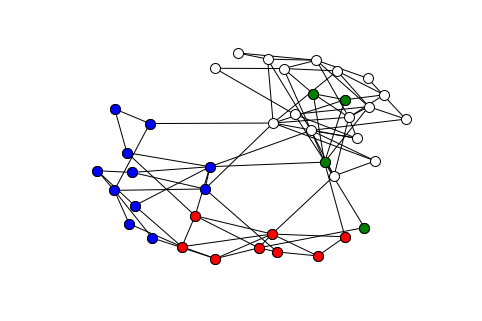# Datasets¶

SNAP = Stanford Network Analysis Project

Graphs with ground-truth communities:

• Social networks $\to$ groups
• Amazon product network $\to$ product categories
• DBLP collaboration network $\to$ conferences, journals

Yang & Leskovec 2012

# Local community detection¶

Classical approach:

• Rank nodes with respect to their "distance" to the seed set
• Evaluate the resulting successive communities
• Select the best one(s)

Clauset 2005, Andersen & Lang 2006

Other approach: Sozio & Gionas 2010

# Outline¶

1. Background
2. Ranking
3. Quality metrics
4. Live examples
5. Future work

# PageRank¶

Ranking = frequency of visits of a random walk

Brin & Page 1998

In :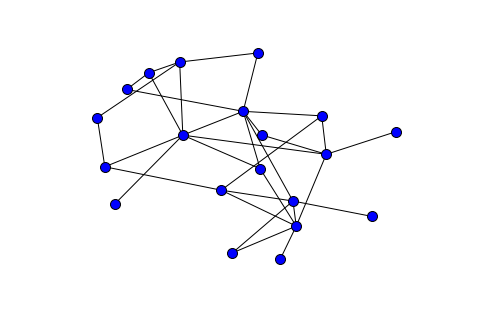# Case of undirected graphs¶

Let $\mu^{(t)}$ be the distribution of the random walk at time $t$: $$\mu^{(t)} = \mu^{(t-1)} P$$ where $P$ is the transition matrix $$P_{ij} = \frac{A_{ij}}{d_i}$$

Limiting distribution: $$\mu^{(t)} \to \mu \propto d$$

# Case of directed graphs¶

What about sinks / absorbing sets?

In :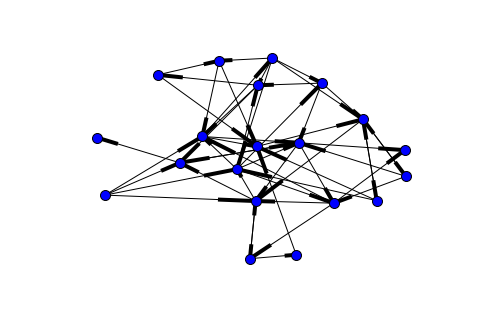# Sinks¶

Modified transition matrix: $$P_{ij} = \left\{ \begin{array}{ll} \frac{A_{ij}}{d^+_i} & \text{if } d^+_i>0\\ \frac 1 n & \text{otherwise} \end{array} \right.$$

# Absorbing sets¶

Damping factor $\alpha \in (0,1)$:

• Walk with probability $\alpha$
• Teleport with probability $1-\alpha$

Default value $\alpha = 0.85$

Walking distance geometric with mean $\frac \alpha{1-\alpha}\approx 5.7$

# Dynamics¶

Let $p^{(t)}$ be the distribution of the random walk at time $t$: $$p^{(t)} = (1-\alpha)p^{(0)} + \alpha p^{(t-1)}P$$ Limiting distribution: $$p^{(t)}\to p = (1-\alpha)\sum_{t=0}^{+\infty}\alpha^t \mu^{(t)}$$

• $\alpha \to 1$ (long paths): $p\to \mu^{(\infty)}$
• $\alpha \to 0$ (short paths): $p\to \mu^{(0)}$ (uniform distribution)

# Revisiting PageRank¶

Idea: In a directed graph, two nodes are "close" if they have many successors in common $\to$ co-citation graph

The weight between $i$ and $j$ is the number of co-citations: $$w_{ij} = \sum_k {A_{ik}A_{jk}}$$ The weight of node $i$ is: $$w_i =\sum_j w_{ij} = \sum_{k}A_{ik} d^-_k$$

# Normalized weights¶

Contribution of each co-citation $k$ normalized by its number of citations: $$w'_{ij} = \sum_k \frac{A_{ik}A_{jk}}{d^-_k}$$ Weight of node $i$ is: $$w'_i =\sum_j w'_{ij} = \sum_{k}A_{ik} = d^+_i$$

A random walk in the co-citation graph corresponds to a forward-backward random walk in the original graph ($\approx$ HITS algorithm)

# Removing self-loops¶

Usual weights: $$\forall i\ne j,\ w_{ij} = \sum_k {A_{ik}A_{jk}} \Longrightarrow w_i = \sum_{j\ne i }w_{ij} = \sum_k A_{ik} (d^-_k - 1)$$ Normalized weights: $$\forall i\ne j,\ w'_{ij} = \sum_k \frac{A_{ik}A_{jk}}{d^-_k} \Longrightarrow w'_i = \sum_{j\ne i }w'_{ij} = \sum_k A_{ik} \frac{d^-_k - 1}{d_k^-}$$ $\to$ non-backtracking forward-backward random walk in the original graph

# Personalized PageRank¶

Damping factor $\alpha\in (0,1)$:

• Start from the seed set
• Walk with probability $\alpha$
• Teleport to the seed set with probability $1-\alpha$

Walking distance geometric with mean $\frac \alpha{1-\alpha}\approx 5.7$

# Dynamics¶

Let $p^{(t)}$ be the distribution of the random walk at time $t$: $$p^{(t)} = (1-\alpha)p^{(0)} + \alpha p^{(t-1)}P$$ Limiting distribution: $$p^{(t)}\to p = (1-\alpha)\sum_{t=0}^{+\infty}\alpha^t \mu^{(t)}$$

• $\alpha \to 1$ (long paths): $p\to \mu^{(\infty)}$
• $\alpha \to 0$ (short paths): $p\to \mu^{(0)}+ \alpha \mu^{(1)}+\alpha^2 \mu^{(2)}+\ldots$

# LexRank¶

• Start from the seed set
• For $k = 1, 2,\ldots$, rank the $k$-hop neighbors after $k$ jumps of the random walk

If the graph is directed, use the co-citation graph!

# Outline¶

1. Background
2. Ranking
3. Quality metrics
4. Live examples
5. Future work

# Conductance¶

The conductance of a community $C$ is: $$\phi(C) = \frac{\sum_{i\in C, j\not\in C} A_{ij}}{\sum_{i\in C, j\in V} A_{ij}}= \frac{\sum_{i\in C} d_i^{\rm out}}{\sum_{i\in C} d_i}$$ This is the probability that a random walk starting from $C$ in steady state leaves $C$ in one jump: $$\phi(C) = \frac{\sum_{i\in C,j\not \in C} d_i P_{ij}}{\sum_{i\in C} d_i}$$ A "good" community has a low conductance

# Strength¶

The strength of a community $C$ is: $$\sigma(C) = 1-\phi(C) = \frac{\sum_{i\in C, j\in C} A_{ij}}{\sum_{i\in C, j\in V} A_{ij}} = \frac{\sum_{i\in C} d_i^{\rm in}}{\sum_{i\in C} d_i}$$ This is the probability that a random walk starting from $C$ in steady state stays in $C$ in one jump: $$\sigma(C) = \frac{\sum_{i\in C,j \in C} d_i P_{ij}}{\sum_{i\in C} d_i}$$ A "good" community is strong in the sense that $\sigma(C)\ge \mu(C)$

# Modularity¶

The modularity is related to the average strength of the communities: $$Q = \sum_{C} \mu(C)(\sigma(C)-\mu(C))$$

Chang et. al. 2015

# Normalized conductance¶

The normalized conductance of a community $C$ is: $$\phi'(C) = \frac{\sum_{i\in C, j\not\in C} A_{ij}/d_i}{\sum_{i\in C, j\in V} A_{ij}/d_i} = \frac 1 {|C|}\sum_{i\in C} \frac{d^{\rm out}_{i}}{d_i}$$ This is the probability that a random walk starting from $C$ leaves $C$ in one jump: $$\phi'(C) = \frac 1{|C|} \sum_{i\in C,j\not \in C} P_{ij}$$

# Normalized strength¶

The normalized strength of a community $C$ is: $$\sigma'(C) = 1-\phi'(C) = \frac{\sum_{i\in C, j\in C} A_{ij}/d_i}{\sum_{i\in C, j\in V} A_{ij}/d_i} = \frac 1 {|C|}\sum_{i\in C} \frac{d^{\rm in}_{i}}{d_i}$$ This is the probability that a random walk starting from $C$ stays in $C$ in one jump: $$\sigma'(C) = \frac 1{|C|} \sum_{i\in C,j\in C} P_{ij}$$ A "good" community is strong in the sense that $\sigma'(C)\ge |C|/n$

# Normalized modularity¶

The normalized modularity is related to the average normalized strength of the communities: $$Q' = \sum_{C} \frac {|C|}n(\sigma'(C)-\frac {|C|}n)$$ We get: $$Q' = \frac 1 {n}\sum_{i,j}\frac{A_{ij}}{d_i}\delta_{ij} - \frac 1{n^2} \sum_{i,j}\delta_{ij}=\frac 1 {n}\sum_{i}\frac{d^{\rm in}_{i}}{d_i} - \frac 1{n^2} \sum_{i,j}\delta_{ij}$$

# Outline¶

1. Background
2. Ranking
3. Quality metrics
4. Live examples
5. Future work

# Approach: directional ranking¶

Input: seed node $s$

For each neighbor $u$ of $s$:

• Rank nodes for the seed set $S=\{s,u\}$
• Compute the normalized strength of the resulting successive communities

# Example: Wikipedia¶

In :
s = inv_page['Donald Trump']
top_pages(s)

Donald Trump
0 Mitt Romney
1 Tim Pawlenty
2 Ann Coulter
3 John McCain presidential campaign, 2008
4 United States House of Representatives elections, 2006
5 Manhattan
6 John McCain
7 CNN
8 United States cable news
9 Michele Bachmann
10 Michael Bloomberg
11 Newt Gingrich
12 Hillary Rodham Clinton
13 Pat Boone
14 The Rush Limbaugh Show
15 Larry King Live
16 Rudy Giuliani
17 Barack Obama citizenship conspiracy theories
18 112th United States Congress
19 Gary Johnson


# Example: Wikipedia¶

In :
direction = 

In :
pagerank_score = simple_detection(s,direction,algo="pagerank")
lexrank_score = simple_detection(s,direction,algo="lexrank")
lexrank_star_score = simple_detection(s,direction,algo="lexrank_star")
plot(pagerank_score,label="PageRank")
plot(lexrank_score,label="LexRank")
plot(lexrank_star_score,label="LexRank*")
xlabel("Community")
ylabel("Normalized strength")
legend(bbox_to_anchor=(1.4, 1))
show()

Donald Trump, Hillary Rodham Clinton, United States, Barack Obama, Republican Party (United States), Democratic Party (United States), New York City, President of the United States, George W. Bush, John McCain,
Donald Trump, Hillary Rodham Clinton, Ted Kennedy, Democratic Party (United States), First inauguration of Barack Obama, John McCain, United States, Joe Biden, John Kerry, History of the United States Democratic Party,
Donald Trump, Hillary Rodham Clinton, John McCain, Mitt Romney, Ted Kennedy, Bill Clinton, Joe Biden, Presidency of Bill Clinton, Ann Coulter, 111th United States Congress,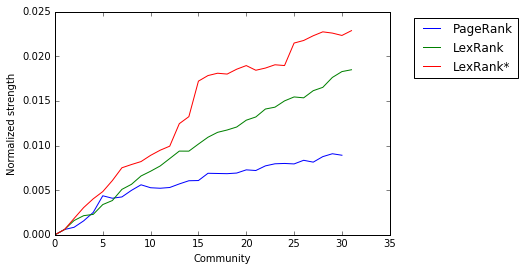# Example: Wikipedia¶

In :
direction = 

In :
pagerank_score,u_pagerank_score = detection(s,direction,algo="pagerank")
lexrank_score,u_lexrank_score = detection(s,direction,algo="lexrank")
lexrank_star_score,u_lexrank_star_score = detection(s,direction,algo="lexrank_star")
figure(figsize=(12, 4))
subplot(121)
plot(u_pagerank_score,label="PageRank")
plot(u_lexrank_score,label="LexRank")
plot(u_lexrank_star_score,label="LexRank*")
xlabel("Community")
ylabel("Strength")
subplot(122)
plot(pagerank_score,label="PageRank")
plot(lexrank_score,label="LexRank")
plot(lexrank_star_score,label="LexRank*")
xlabel("Community")
ylabel("Normalized strength")
legend(bbox_to_anchor=(1.4, 1))
show()

Paris, French Revolution, France, Napoleon, Louis XVI of France, Reign of Terror, Departments of France, National Convention, Ancien Régime, National Constituent Assembly,
Paris, French Revolution, France, History of France, Napoleon, Liberalism, History of Europe, July Monarchy, Louis XVI of France, Maximilien de Robespierre,
Paris, French Revolution, France, History of France, Maximilien de Robespierre, Napoleon, Louis XVI of France, Gilbert du Motier, Marquis de Lafayette, July Monarchy, Georges Danton,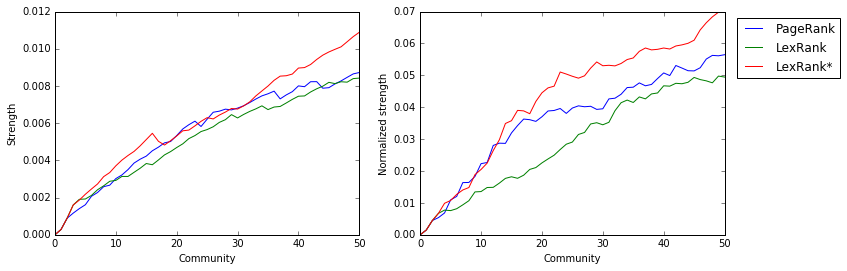# Outline¶

1. Background
2. Ranking
3. Quality metrics
4. Live examples
5. Future work

# Future work¶

Improved algorithms through

Application to data analysis $\to$ similarity graph